# Team:Dundee/Project/PP1Capacities

iGEM Dundee 2013 · ToxiMop

Microcystin’s toxic action lies in its ability to bind to and thus deactivate the human Protein Phosphatase-1 (PP1). Microcystin covalently binds to PP1 in a one-to-one relationship. Therefore, the larger the quantity of PP1 produced within a given chassis, the greater the "mopping" potential .

The two options were explored for our bacterial mop: (i) to export PP1 to the periplasm of E. coli and (ii) to export and then bind PP1 to the membrane surface of B. subtilis. To provide a crude estimate of "maximum mop efficacy" i.e. maximal PP1-binding potential, we investigated the packing properties of each chassis option.

## Theory

When considering the maximum number of PP1 molecules that cold be located into the periplasm of E. coli or onto the membrane surface of B. subtilis, consideration for wasted space must be taken into account. This wasted space arises due to the physical configuration of PP1.

Before beginning such analyses, assumptions must be made regarding the shape and form of the PP1 molecules. To best describe the protein shape, without unnecessary over complication, PP1 was approximated as a sphere with a diameter equal to the width of the minimal osculatory cubic enclosure of the molecule. This approximation allows for the possibility of random orientations of the PP1 molecules and therefore gives an average result. The radius of PP1 is thus calculated as ~9.1nm.

To calculate the available volume within E. coli or B. subtilis cells, both chassis were approximated as cylindrical bodies with hemispherical ends. Furthermore, the dimensions of E. coli and B. subtilis cells were taken as being approximately equivalent and were based upon the known dimensions of E. coli: 0.5 μm in width by 2 μm in length  .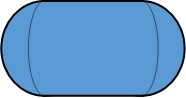The equations describing the volume of spheres of radius r and cylinder of radius r and height h are given by :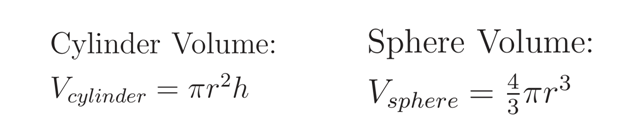Making use of these equations, the volume of an E. coli cell can be calculated as the volume of a cylinder of radius 0.25μm and height 1.5μm and the volume of a sphere of radius 0.25μm (the two hemispheres can be brought together and calculated as a single sphere).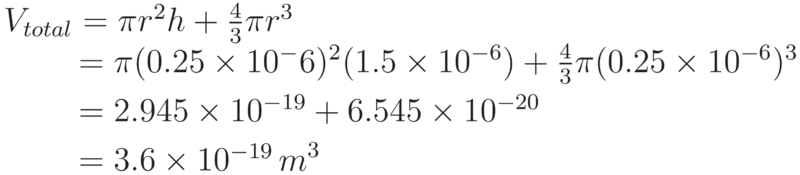## E. coli

For E. coli with a periplasmic width of 21nm, the periplasm volume can be calculated by considering the cell as two parts.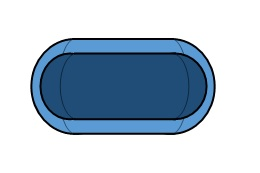To calculate the periplasmic volume of E. coli, we next needed to calculate the volume enclosed by the inner membrane. This is again taken to be a cylinder now of radius 0.25-0.021 = 0.229 μm and length of 1.5μm. The two flanking hemispheres in this case have a radius 0.229μm. Therefore the volume enclosed by the inner membrane is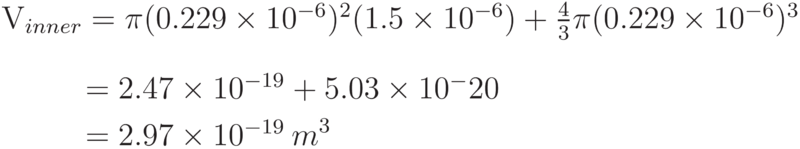Therefore, the volume of the periplasm in E. coli is calculated to be: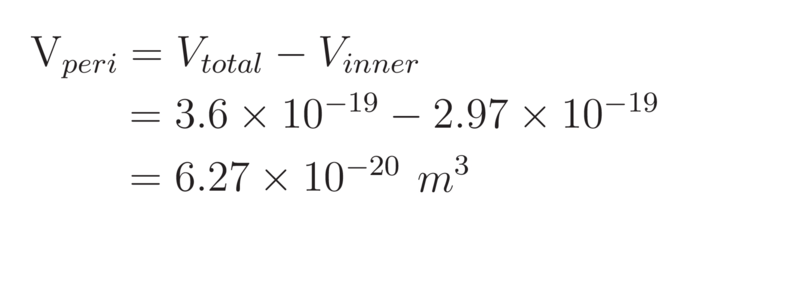To determine the maximum number of PP1 molecules that could be packed into the periplasm, we determined the volume of a single PP1 molecule. This volume can be taken as that of a sphere of diameter 9.1nm. However, when considering the volume that a single molecule will occupy, the space in between molecules must also be considered. To take account of this wasted space, a cubic approximation was made: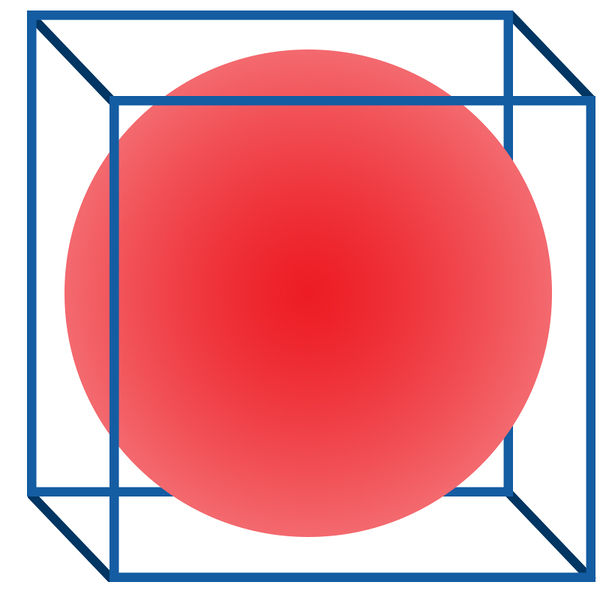Thus, the volume of a PP1 molecule is assumed to be: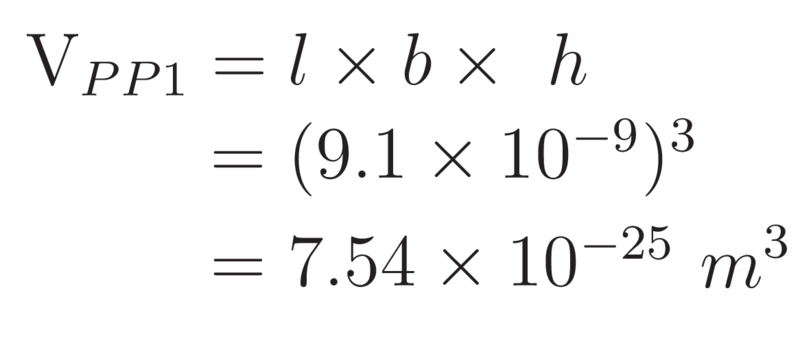Hence, the maximum number of PP1 molecules that could be packed into the periplasm of E. coli can be approximated by: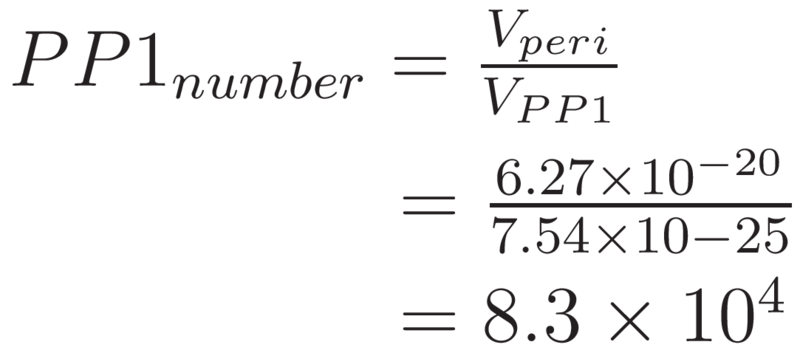## B. subtilis

When considering the maximal packing of PP1in B. subtilis we recall that it is a Gram-positive cell and so the PP1 molecules must be adhered in a monolayer to the surface of the cell membrane.

In packing PP1 on the surface of the membrane, the number of PP1 molecules that can be placed “side-by-side” along the height of the membrane and around the circumference of the membrane was calculated.The number of PP1 molecules able to be stacked along the length of the cylindrical centrepiece of the cell (excluding the hemispheres) and around the circumference of the cylinder top: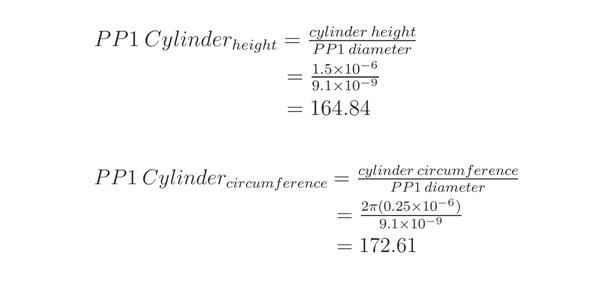Multiplying the above results gives the total number of PP1 molecules which can occupy the surface of the cylindrical midsection of the bacteria.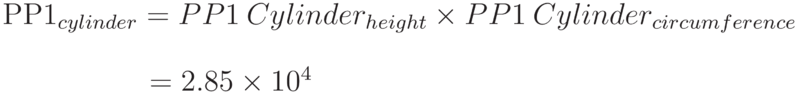The number of PP1 molecules that could occupy the hemispherical ends can be calculated by approximating the hemispherical ends as discs. This approximation is appropriate due to the smaller contribution at these ends.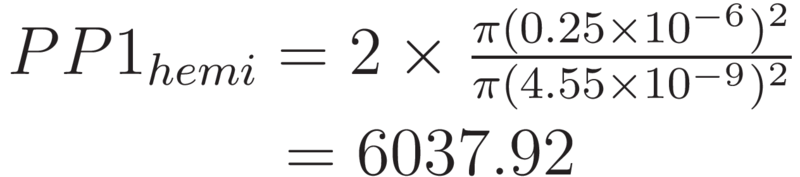Thus, the total number of PP1 that could occupy the surface of B. subtilis can be calculated as the sum of the above.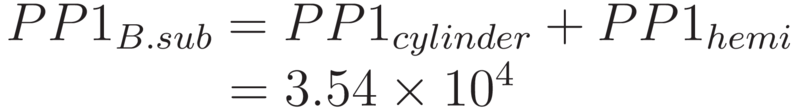## Conclusion

Our calculations show the maximum number of PP1 molecules that could fit on the surface of B. subtilis is approximately 35,000. For E. coli, the PP1 is contained within the periplasm. This periplasmic volume could potentially contain many more PP1 molecules (83 000) than could be anchored to the membrane of B. subtilis. Therefore, E. coli has the greater potential to be a more efficient mop. Consequently, using E. coli would mean that less bacterial mops would be required to remove a given quantity of microcystin. Using this approximation, we calculated that the number of E. coli mops required to clean a toxic level of microcystin in a litre of water to be 2.2x109 cells.

Having shown that E. coli has the potential to be the more efficient mop, this result was presented to the wet team in Week 1. The wet lab continued to explore the use of both species, but later in the project it was found that there were issues in exporting PP1 to the surface of B. subtilis. Hence our team focussed on E. coli as the chassis for the bacterial mop, in line with the original modelling prediction.